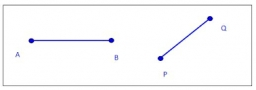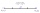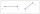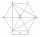# Midpoint 6

For line segment length is given: FM=8a+1, FG=42. Point M is the midpoint of FG. Find unknown a.

a =  2.5

### Step-by-step explanation:

8•a+1 = 42/2

16a = 40

a = 5/2 = 2.5

Our simple equation calculator calculates it.Did you find an error or inaccuracy? Feel free to write us. Thank you!Tips to related online calculators
Looking for help with calculating arithmetic mean?
Looking for a statistical calculator?
Do you have a linear equation or system of equations and looking for its solution? Or do you have a quadratic equation?
Do you want to convert length units?

#### You need to know the following knowledge to solve this word math problem:

We encourage you to watch this tutorial video on this math problem:

## Related math problems and questions:

• MG=7x-15,Length of lines MG = 7x-15 and FG = 33 Point M is the midpoint of FG. Find the unknown x.
• Find midpointFM=5y+13, MG=5-3y, FG=? M is the midpoint of FG. Use the given information to find the missing measure or value.
• Midpoint 5FM=3x-4, MG=5x-26, FG=? Point M is the midpoint of FG. Use the given information to find the missing measure or value.
• Midpoint 4If the midpoint of a segment is (6,3) and the other end point is (8,-4) what are thw coordinate of the other end?
• Coordinates of midpointIf the midpoint of the segment is (6,3) and the other end is (8,4) what are the coordinate of the other end?
• Midpoint of segmentPoint A has coordinates [4; -11] and the midpoint of the segment AB is the point [17; -7]. What are the coordinates of point B?
• Points on line segmentPoints P & Q belong to segment AB. If AB=a, AP = 2PQ = 2QB, find the distance: between point A and the midpoint of the segment QB.
• The midpointThe midpoint of (2, 5) and (8, y) is (5, -1). Find the line equation in slope-intercept form.
• Find the 3Find the distance and midpoint between A(1,2) and B(5,5).
• Points OPQPoint P is on line segment OQ. Given OP = 6, OQ = 4x - 3, and PQ = 3x, find the numerical length of OQ.
• Line segmentCut a line segment of 15 cm into two line segments so that their lengths are in ratio 2:1. What length will each have?
• Line segmentFor the line segment whose endpoints are L[-1, 13] and M[18, 2], find the x and y value for the point located 4 over 7 the distance from L to M.
• SegmentsWhich of the pairs of numbers on the number line encloses the longest segment: ?
• Line segmentThe 4 cm long line segment is enlarged in the ratio of 5/2. How many centimeters will measure the new line segment?
• Center of line segmentCalculate the distance of the point X [1,3] from the center of the line segment x = 2-6t, y = 1-4t ; t is from interval <0,1>.
• The coordinatesThe coordinates (5, 2) and (-6, 2) are vertices of a hexagon. Explain how to find the length of the segment formed by these endpoints. How long is the segment?
• Trapezoid MO-5-Z8ABCD is a trapezoid that lime segment CE is divided into a triangle and parallelogram, as shown. Point F is the midpoint of CE, DF line passes through the center of the segment BE, and the area of the triangle CDE is 3 cm2. Determine the area of the trape